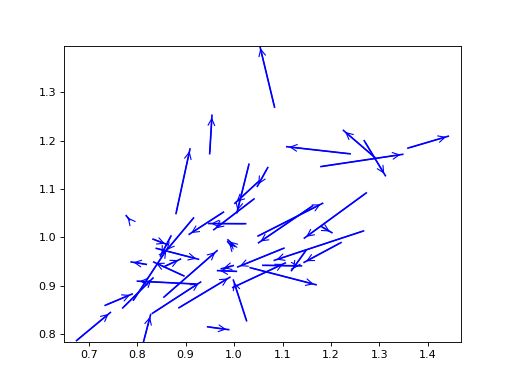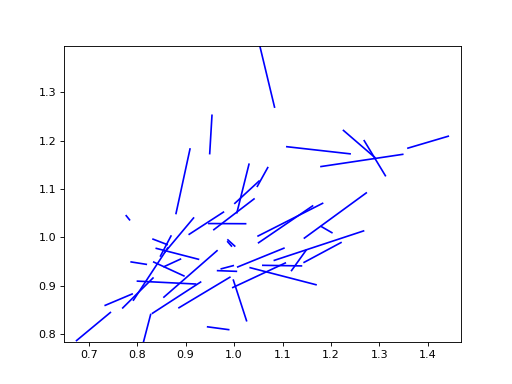splot.giddy.dynamic_lisa_vectors¶

splot.giddy.dynamic_lisa_vectors(rose, ax=None, arrows=True, **kwargs)[source]

Plot vectors of positional transition of LISA values in Moran scatterplot

Parameters
rosegiddy.directional.Rose instance

A Rose object, which contains (among other attributes) LISA values at two points in time, and a method to perform inference on those.

axMatplotlib Axes instance, optional

If given, the figure will be created inside this axis. Default =None.

arrowsboolean, optional

If True show arrowheads of vectors. Default =True

**kwargskeyword arguments, optional

Keywords used for creating and designing the matplotlib.pyplot.plot() Note: ‘c’ and ‘color’ cannot be passed when attribute is not None.

Returns
figMatplotlib Figure instance

Figure of dynamic LISA vectors

axmatplotlib Axes instance

Axes in which the figure is plotted

Examples

>>> import geopandas as gpd
>>> import pandas as pd
>>> from libpysal.weights.contiguity import Queen
>>> from libpysal import examples
>>> import numpy as np
>>> import matplotlib.pyplot as plt
>>> from giddy.directional import Rose
>>> from splot.giddy import dynamic_lisa_vectors

get csv and shp files

calculate relative values

>>> for year in range(1969, 2010):
...     income_table[str(year) + '_rel'] = (
...         income_table[str(year)] / income_table[str(year)].mean())

merge to one gdf

>>> gdf = df.merge(income_table,left_on='STATE_NAME',right_on='Name')

retrieve spatial weights and data for two points in time

>>> w = Queen.from_dataframe(gdf)
>>> w.transform = 'r'
>>> y1 = gdf['1969_rel'].values
>>> y2 = gdf['2000_rel'].values

calculate rose Object

>>> Y = np.array([y1, y2]).T
>>> rose = Rose(Y, w, k=5)

plot

>>> dynamic_lisa_vectors(rose)
>>> plt.show()customize plot

>>> dynamic_lisa_vectors(rose, arrows=False, c='r')
>>> plt.show()

(png, hires.png, pdf)